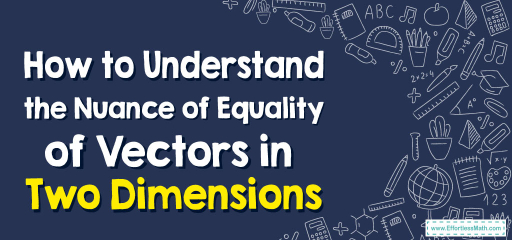# How to Understand the Nuance of Equality of Vectors in Two Dimensions

A vector is a mathematical tool that captures the dual concepts of size and direction, making it indispensable in numerous applications ranging from physics to computer science.## Step-by-step Guide to Understand the Equality of Vectors in Two Dimensions

Here is a step-by-step guide to understand the equality of vectors in two dimensions:

### Step 1: Embarking on the Basics

• Vectors Defined: In the realm of mathematics, a vector is an articulate representation encapsulating both magnitude (how long?) and direction (which way?).
• Two-dimensional Dance: For our present discourse, we’ll delve into a plane where each vector gracefully dances with two components: along the $$x$$-axis (horizontal) and the $$y$$-axis (vertical).

### Step 2: The Algebraic Presentation

• Formulation: A vector in this two-dimensional setting is typically expressed as $$v=(x,y)$$, where $$x$$ and $$y$$ are scalars signifying the components.
• Visualization: Envision a Cartesian plane. Place the vector’s tail at the origin and its tip at the coordinate $$(x, y)$$.

### Step 3: Establishing the Idea of Equality

• Fundamental Understanding: Two vectors are said to be equal if, and only if, they have identical magnitudes and identical directions.
• Algebraic Translation: For two vectors $$a=(x_1​,y_1)$$ and $$b=(x_2​,y_2​)$$, their equality is affirmed when $$x_1​= \ x_2$$ and $$y_1​= \ y_2$$​.

### Step 4: Visual Corroboration in the Coordinate System

• Graphical Symmetry: On plotting, equal vectors, irrespective of their initial position, will always overlay perfectly upon each other when superimposed.
• Directional Concord: Their arrowheads point unwaveringly in the same direction, and their lengths are mirror reflections of one another.

### Step 5: Distinguishing Position vs. Equality

• Ubiquity of Position: While a vector may start from any point in the plane, its essence remains rooted in its magnitude and direction.
• Clarification: Vectors with matching components might originate from different positions in the plane but are, by definition, equal. Their starting point is immaterial to their inherent equality.

### Step 6: Navigating Potential Pitfalls

• Avoid Confusion: Do not mistake equal magnitude as indicative of equal vectors. Direction plays an equally pivotal role.
• Vectorial Identity: Even a slight deviation in either component results in a different vector. Precision is paramount.

### Step 7: Exploring Broader Implications

• Beyond Two Dimensions: This foundational understanding of vector equality seamlessly extends into three dimensions and even abstract vector spaces.
• Physical Resonance: In physics, equal vectors might denote forces acting in perfect harmony or velocities moving objects in synchronized motion.

To fathom the equality of vectors in two dimensions is to delve deeply into a symphony of algebraic precision and geometric artistry. By mastering this, you are not only becoming adept in mathematics but also appreciating the subtle harmonies that govern the universe. Embrace the journey, and let the vectors guide you through the intricacies of space and logic.

### Examples:

Example 1:

Are the vectors $$C=(3,7)$$ and $$D=(3,8)$$ equal?

Solution:

To determine if vectors $$C$$ and $$D$$ are equal, we need to compare their respective components:

• $$x$$-component of $$C=3$$
• $$x$$-component of $$D=3$$
• $$y$$-component of $$C=7$$
• $$y$$-component of $$D=8$$

Since the $$x$$-components are the same but the $$y$$-components are different $$(7 ≠ 8)$$, the vectors $$C$$ and $$D$$ are not equal.

Example 2:

Are the vectors $$E=(−2,5)$$ and $$F=(−2,5)$$ equal?

Solution:

To ascertain the equality of vectors $$E$$ and $$F$$, let’s contrast their individual components:

• $$x$$-component of $$E = -2$$
• $$x$$-component of $$F = -2$$
• $$y$$-component of $$E = 5$$
• $$y$$-component of $$F = 5$$

Given that both the $$x$$-components and the $$y$$-components match exactly ($$-2 = -2$$ and $$5 = 5$$), the vectors $$E$$ and $$F$$ are equal.

### What people say about "How to Understand the Nuance of Equality of Vectors in Two Dimensions - Effortless Math: We Help Students Learn to LOVE Mathematics"?

No one replied yet.

X
30% OFF

Limited time only!

Save Over 30%

SAVE $5 It was$16.99 now it is \$11.99